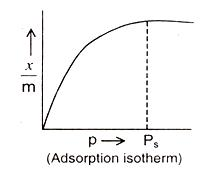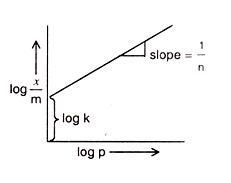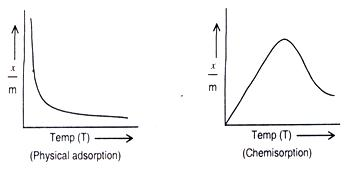Click to Chat

1800-1023-196

+91-120-4616500

CART 0

• 0

MY CART (5)

Use Coupon: CART20 and get 20% off on all online Study Material

ITEM
DETAILS
MRP
DISCOUNT
FINAL PRICE
Total Price: Rs.

There are no items in this cart.
Continue ShoppingAdsorption of Gases on Solids

Table of Content

Nature of Gas

Nature of Solid

Specific Area of Solid

Pressure of a Gas

Temperature

Activation of Solid

Related Resources

The extent of adsorption of a gas on the surface of a solid depends on the following factors:

Nature of gas

Nature of solid

Specific area of solid

Pressure of gas

Temperature

Activation of solid

Nature of Gas

Since physical adsorption is non-specific in nature, any gas will be adsorbed on the surface of a solid to some extent or other. However, under any given conditions of temperature and pressure, easily liquefiable gases such as NH3, CH4, HCI, CI2, SO2, CO etc. are adsorbed more than permanent gases like H2, O2, N2 etc. Chemisorption is specific in nature. Therefore, only those gases will be adsorbed which form chemical bonds with it.

Nature of Solid

Activated charcoal is the most common adsorbent for easily liquefiable gases. Poisonous gases such as CH4 and CO fall in this group. Therefore, it is used in gas masks. Other gases such as O2, H2 and N2 adsorb more on metals such as Ni, Pt and Pd.

Specific Area of Solid

Specific area of an adsorbent is the surface area available for adsorption per gm of adsorbent. Greater the specific area of an adsorbent, greater will be the adsorption. The specific area of an adsorbent can be increased by making the surface rough. The pores must be large enough to allow penetrations of gas molecules.

Pressure of a Gas

As physical adsorption is reversible, it is accompanied by decrease in pressure. Therefore, it is expected that at a given temperature the extent of adsorption will increase with the increase of pressure of the gas.

The extent of adsorption is measured as x/m where m is the mass of adsorbent and x that of adsorbate.If the physical adsorption is limited to unimolecular layer, the plot of x/m vs. equilibrium pressure at a constant temperature is as shown.

It is evident from the graph that at a certain pressure the adsorption reaches a maximum value i.e. the adsorption becomes saturated and the corresponding pressure is called saturation pressure (Ps). Beyond this pressure the adsorption remains constant.
At low pressures : x/m varies linearly with p

or

At high pressures :  x/m is independent of p

At intermediate pressures:  The variation of x/m vs p can be expressed as

where
n > 1.
or

or

Comparing the above given equation with equation of straight line

y = mx + c

we know that, if we plot log p vs log x/m, we would get a straight line with slope equal to 1/n and intercept log k.This is called Freundlich adsorption isotherm.

Refer to the following video for Freundlich adsorption isotherm.

Example

Question:

Apply Freundlich adsorption isotherm, calculate amount of acetic acid adsorbed by 1 kg of blood charcoal at 25 oC from 5% vinegar solution( mass/volume). Given that if concentration is expressed in molarity , x/m is mass of solute adsorbed per gram of adsorbent, then k = 0.160 and n = 2.32.

Solution:

According to freundlich adsorption isotherm, x.m = kc1/n…..(i)

5% vinegar means 5 g of acetic acid are present in 100 mL of the solution.

Molar mass of acetic acid (CH3COOH) = 60 g mol-1.

5 g of Acetic acid = 5/60 mol

1000 mL of the solution will contain acetic acid = (5/60)(1/100)(1000) = 0.837 mol.

i.e. C = 0.837 mol L-1

x/m = 0.160 (0.837)1/2.32

solving the above equation

x/m = 0.1481 g

so, amount adsorbed by 1 kg of charcoal = 148.1 g

Temperature

As adsorption is accompanied by release of heat energy, so in accordance with Le-Chatelier’s principle, the increase of temperature should decrease the extent of adsorption. This has indeed been found to be so. A plot of x/m vs. temperature at constant pressure is called adsorption isobar.

In the case of physical adsorption x/m decreases with increase of temperature. However, in the case of chemisorption x/m initially increases with temperature and then decreases as shown below.

The initial increase is due to the fact that chemisorptions require activation energy.Activation of Solid

Activation of adsorbent means increasing its adsorbing power. This is increased by increasing specific area either by making the surface rough or by breaking the solid into smaller particles. But care must be taken so that particles do not become very small, otherwise the inter-particle spaces will be too small to allow penetration of gas molecules.A strongly adsorbable substance can easily displace a weakly adsorbed substance. For example, on the surface of activated charcoal, gases such as O2, N2 etc. are already adsorbed. But when charcoal comes in contact with poisonous gases such as CH4, CO which are strongly adsorbable, O2 and N2 get displaced.

If a mix of gases is allowed to adsorb on a particular adsorbent, the strongly adsorbable adsorbate adsorbs to a greater extent than its partial pressure indicates. For example, moisture though present in small proportion in air is strongly adsorbed by silica gel. Charcoal adsorbs poisonous gases even though they are present in small concentration in air.

Some solids are capable of adsorbing certain solutes from the solution. This property is made use of in adsorbing colouring matter from solutions of organic substances.

Raw cane juice is decolourised by activated charcoal. Similarly, charcoal adsorbs oxalic and acetic acid dyes from their aqueous solutions.

Freundlich adsorption isotherm is also applicable to solutions by replacing the pressure term by the concentration (C) of adsorbates in solutions. The relationship is modified as follows .Taking logarithm, it becomes

log x/m = logk + 1/n logC

A graph between log (x/m) and log C is a straight line for small ranges of  concentrations.

Another isotherm derived by Langmuir considers the formation of a mono-molecular layer of adsorbate on adsorbent. It is known as Lamuir adsorption isotherm and is based on the following points :

It takes place on the surface of solids till a unimolecular layer is formed.

Adsorption is result of condensation of adsorbent molecules on solid surface and their evaporation.

At equilibrium, the rate of condensation of adsorbate molecules on solid surface and the rate of  their evaporation becomes equal.

Based on these aspects, Lagmuir derived the expression given below:The values of constants “a” and “b” depends upon the nature of adsorbate, nature of solid adsorbent, and temperature. “p” is equilibrium pressure

Lagmuir theory has limitations. This theory of unimolecular adsorption is valid only at low pressure and high temperature.

As the pressure is increases or temperature is lowered, additional layer starts forming . This leads to multilayer adsorption.Question 1: Rate of adsorption ioncreases with

a. increase in temperature

b. increase in surface area of adsorbent

c. decrease in concentration of adsorbate

d. decrease in pressure

Question 2: Which of the following equations correctly represents the Lamuir adsorption isotherm?

a. log x/m = logk + 1/n logC

b. log m/x = logk + n logC

c.

d.

Question 3: The basic principle behind the activation of charcoal is ..

a. increasing the reactivity of charcoal

b. increasing the surface area of charcoal

c. increasing the temperature

d. increasing the purity of charcoal

Question 4: Lagmuir theory of unimolecular adsorption is valid only at

a. low pressure and high temperature

b. high pressure and low temperature

c. low pressure and low temperature

d. high pressure and high temperatureQ.1

Q.2

Q.3

Q.4

b

a

b

a

Related Resources

You can also have a look at past year papers of IIT JEE

You can also refer to adsorption### Course Features

• 731 Video Lectures
• Revision Notes
• Previous Year Papers
• Mind Map
• Study Planner
• NCERT Solutions
• Discussion Forum
• Test paper with Video Solution